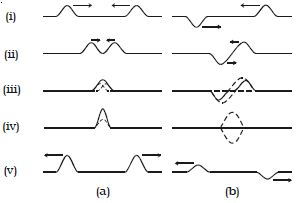# Explain Superposition Principle

When two waves travel in a medium simultaneously in such a way that each wave represents its separate motion, then the resultant displacement at any point at any time is equal to the vector sum of the individual displacements of the waves. This principle is illustrated by means of a slinky in the Fig. (a).

1. In the figure, (i) shows that the two pulses pass each other.
2. In the figure, (ii) shows that they are at some distance apart
3. In the figure, (iii) shows that they overlap partly
4. In the figure, (iv) shows that resultant is maximumFig. (b) illustrates the same events but with pulses that are equal and opposite.

If Y1 and Y2 are the displacements at a point, then the resultant displacement is given by

Y = Y1 + Y2.

If  Y1 = Y2 = a, and if the two waves have their displacements in the same direction. then │y│ = a + a = 2a

If the two waves have their displacements in the opposite direction, then │y│ = a + (-a) = 0

The principle of superposition of waves is applied in wave phenomena such as interference, beats and stationary waves.